Mathematics

# Carl Friedrich Gauss

534 views

 1 Introduction 2 Impact of Gauss in Mathematics 3 Awards & Honors 4 Lasting Legacy 5 FAQs

07 October 2020

## Introduction

Johann Carl Friedrich Gauss was born on April 30, 1777 within the city of Brunswick, Germany.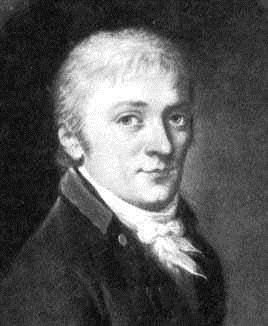His mother was Dorothea Benze and his father was Gebhard Dietrich Gauss. In 1792, age 15, supported by the Duke, Carl entered Caroline College. By the time he was 18, he had completed his degree in mathematics.

## Impact of Gauss in Mathematics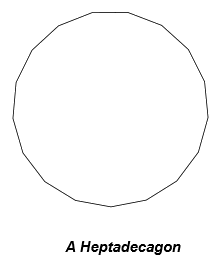The development of a daily 17-sided figure, the heptadecagon, by straightedge and compass alone.

The Ancient Greeks had shown that regular 3, 5, and 15-sided polygons are often constructed using only a straightedge and compass, but had not been ready to discover any longer such shapes.

In fact, Gauss went beyond even the heptadecagon.

He discovered a mathematical formula to seek out all regular polygons which will be constructed using only straightedge and compass – and located 31. Following the 17-sided figure are the 51, 85, 255, 257,….., and 4,294,967,295-sided figures.

### Gauss’s Heliotrope

Gauss invented the heliotrope in 1821. He had become entangled in land surveys for map-making and saw the importance of recording far-separated positions with great accuracy.

The heliotrope may be a mirror that reflects the sun’s rays over very long distances.

Its drawback is it can only be utilized in bright sunshine.

Heliotropes were utilized in land surveys in Germany for over 150 years. They were also used to survey the USA.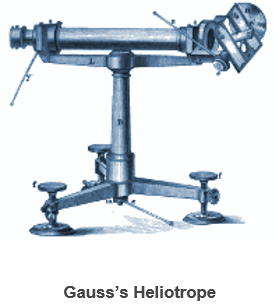#### Electricity and Magnetism

In 1831, Gauss began to use mathematical potential theory to the important world. The 54-year-old mathematician helped the 27-year-old physicist Wilhelm Weber on electricity and magnetism. In 1832, with Weber’s assistance, Gauss administered experiments whose results allowed him to define the earth’s magnetic flux using units of millimeters, grams, and seconds. In other words, he showed the earth’s magnetic flux is often defined using purely mechanical dimensions – mass, length, and time.

The work provided a strong impetus for the utilization of SI units.

### The Telegraph

In 1833, Gauss and Weber invented one among the world’s first telegraph systems. They also invented a binary alphabet code, enabling communication between Weber’s physics building and Gauss’s astronomical observatory about 1.5 miles (2.5 km) apart.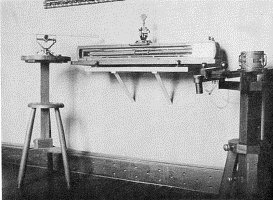In 1833, Gauss and Weber discovered how voltage and current are distributed within the branches of electrical circuits: voltage is governed by the law of conservation of energy, and current by the law of conservation of charge. Gustav Kirchoff rediscovered the laws in 1845, and they now bear his name.

### Gauss’s Law & Gauss’s Law for Magnetism

Gauss research the behavior of electrical and magnetic fields. Using his divergence theorem, which he discovered independently of Joseph-Louis Lagrange, he formulated two laws in 1835.

Gauss’s Law, which relates an electrical field to the distribution of electrical charges that cause it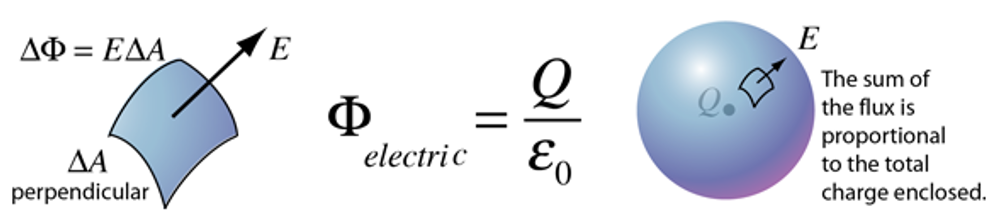Gauss’s Law for Magnetism, which states that magnetic monopoles don't exist

It is written in integral form as ∮B⋅dl=μ0Ienc , where Ienc is the enclosed current and μ0 is a constant.

Written mathematically, these laws form two of the four equations needed to mix the electrical and magnetic fields into one, unified electromagnetic field. The unification was achieved by James Clerk Maxwell in 1864.

## Awards & Honors

The Carl Friedrich Gauss Prize for Applications of Mathematics is a mathematics award, granted jointly by the International Mathematical Union Society. They get its name from the German mathematician Carl Friedrich Gauss. With its premiere in 2006, it's to be awarded every fourth year, at the International Congress of Mathematicians.

The previous laureate was presented with a medal and a cash purse of EUR10,000 funded by the International Congress of Mathematicians 1998 budget surplus.

The official announcement of the prize happened on 30 April 2002, the 225th anniversary of the birth of Gauss. The prize was developed specifically to offer recognition to mathematicians; while mathematicians influence the planet outside of their field, their studies are often not recognized. The prize aims to honor those that have made contributions and effects within the fields of business, technology, or maybe day-to-day life.

As the story goes, when Gauss was a young boy, he was given a question to sum the integers from 1 to 100. Remember that there have been no calculators in those days!

As the other students struggled with this lengthy addition problem, Gauss saw a special way to solve this problem. He listed the primary 50 terms, then listed the second fifty terms in reverse order below the primary set. you'll consider it as he "wrapped" the series back onto itself.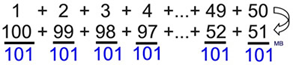Gauss then added the paired values, noticing that the sums were all an equivalent value (101).

Since he had 50 such pairs, he multiplied 101 times 50 and obtained the sum of the integers from 1 to 100 to be 5050.

Now, Gauss's discovery works nicely as long as you've got an Even number of terms in your series.

But what happens to the "wrapped" pairings if the series has 25 terms?

Well, Gauss’s discovery would wish for a touch of tweaking. If the amount of terms is odd, don't split the series in half. Simply list the whole series forward, then list the whole series in reverse and add the pairs. During this situation, you'll have to multiply the sum by the number of pairs then divide by two, since you're actually working with 2 complete series.

By observing the series from BOTH directions simultaneously, Gauss was ready to quickly solve the matter and establish a relationship that we still use today when working with arithmetic series.

Let's generalize what Gauss actually did. Consider the following:

$$a_1 + a_2 + a_3 + . . . + a_n-1 + a_n$$

Let's represent 1+2+3+...+100 in subscripted a notation

$$a_1 + a_n$$

it'll be easier if we elect the primary pair on the left, the addition of the primary and last term, as these terms are usually more readily available.

Gauss multiplied this sum times 50, which is HALF the amount of terms in his sequence. So, we'd like to multiply times half the amount of terms within the sequence, which is represented by n.

Let's generalize what Gauss actually did. Consider the following:

 $$a_1 + a_2 + a_3 + . . . + a_{n-1} + a_n$$ Let's represent 1+2+3+...+100 in subscripted a notation $$a_1 + a_n$$ All of Gauss' make pairs that have a sum of 101. While any pair could be used, it will be easier if we choose the first pair on the left, the addition of the first and last term, as these terms are usually more readily available. \begin{align}\frac{n}{2}(a_1+a_2)\end{align} Gauss multiplied this sum times 50, which is HALF the number of terms in his sequence, and to multiply times half of the number of terms in the sequence, which is represented by n. Is this starting to look familiar? \begin{align}S_n=\frac{n}{2}(a_1+a_2) \end{align} There a formula was, born for the sum of n terms of an arithmetic sequence.

You may see the formula written as:

\begin{align}S_n=\frac{n}{2}(a_1+a_2) \text{ or } S_n=\frac{n(a_1+a_2)}{2}\end{align}

Terms of an arithmetic series are Sum, Sn, of n.

 Example 1 (Even Number of Terms)

Find the sum of two + 4 + 6 + 8 + 10 + 12 + 14 + 16.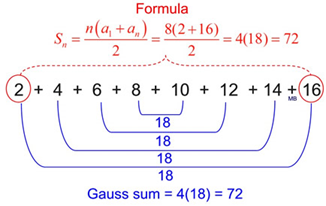Example 2: (Odd Number of Terms)

Find the sum of two + 4 + 6 + 8 + 10 + 12 + 14 + 16 + 18.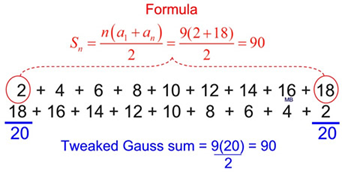Question

An arithmetic series is pairing up terms that always give the same sums. Why?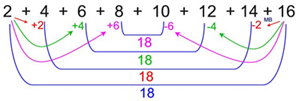If you examine the graphic above, you'll see that if you add the primary and last terms you get 18. If you add the second term to the seventh term you'll also get 18 because you're +2 and -2 away from the primary and last terms. Thus, you've got moved +2 - 2 = 0 away from 18. Remember that in an arithmetic series, the common difference is constant and this pattern of adding and subtracting an equivalent value because the terms are paired will continue. All sums are going to be 18.

## Lasting Legacy

There are many reasons why Carl Friedrich Gauss is still so relevant today. Naturally, some of the problems he could not solve attracted attention, and so you can say that he created a whole field of research. It turns out to have deep connections with the elliptic function theory, too.

In addition, his discovery of the intrinsic concept of curvature enriched the whole study of surfaces and inspired all the work that came after it for generations. Anyone who studies surfaces, from adventurous modern architects to mathematicians, is in his debt. The intrinsic geometry of surfaces extends to the idea of the intrinsic geometry of higher dimensional objects, such as three-dimensional space and four-dimensional space-time. Einstein’s general theory of relativity and all of modern cosmology, including the study of black holes, became possible because Gauss made that breakthrough. And the idea of non-Euclidean geometry, so shocking in its day, made people realize that there can be many kinds of rigorous mathematics, some of which may be more accurate or useful – or just plain interesting – than the ones we thought we knew.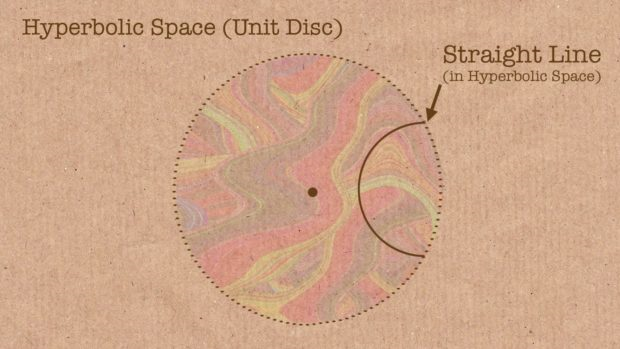## What is Gauss most famous for?

He was probably the greatest mathematician the world has ever known – although perhaps Archimedes, Isaac Newton, and Leonhard Euler also have legitimate claims to the title.

## When was Gauss born?

Johann Carl Friedrich Gauss was born on April 30, 1777, in the city of Brunswick, Germany

## What is Gauss law?

In physics, Gauss's law for magnetism is one of the four Maxwell's equations that underlie classical electrodynamics. It states that the magnetic field B has divergence equal to zero, in other words, that it is a solenoidal vector field. It is equivalent to the statement that magnetic monopoles do not exist.

## What is the conversion formula for Gauss to Tesla?

1 Tesla = 10,000 Gauss

## What is a Gauss Meter used for?

The gauss meter is a reliable measuring device for measuring the existing magnetic field. This gauss meter is suitable for measuring both static/permanent magnet (DC) and alternating magnetic (AC) fields.

Award-winning math curriculum, FREE for a year